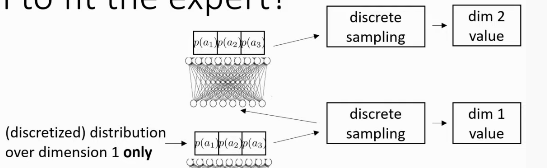# Imitation Learning

## Behavioural Cloning

Behavioural cloning is a fancy name for supervised learning. We collect tuples of actions and observations from demonstrations, and used supervised learning to learn a policy $$\pi_{\theta}(a_t | o_t)$$.

The problem with behavioural cloning is that the errors accumulate, and the state trajectory will change dramatically. When we evaluate the algorithm, can we make $$p_{data}(o_t) = p_{\pi_\theta}(o_t)$$?

### DAgger: Dataset Aggregation

• Goal: collect training data from $$p_{\theta_\pi}(o_t)$$ instead of $$p_{data}(o_t)$$
• how? run $$\pi_\theta (a_t | o_t)$$, but need labels $$a_t$$!
1. train $$\pi_\theta(a_t | o_t)$$ from human data $$\mathcal{D}$$
2. run $$\pi_\theta(a_t|o_t)$$ to get dataset $$\mathcal{D_\pi}$$
3. Ask human to label $$D_\pi$$ with actions $$a_t$$
4. Aggregate: $$\mathcal{D} \leftarrow \mathcal{D} \cup \mathcal{D}_\pi$$
• Problem: have to ask humans to label large datasets iteratively, and can be unnatural (resulting in bad labels)
• Behavioural cloning may still work when we model the expert very accurately (no distributional “drift”)

### Why might we fail to fit the expert?

1. non-Markovian behaviour

1. Our policy assumes that the action depends only on the current observation.
2. Perhaps a better model is to account for all observations.
3. Problem: history exacerbates causal confusion (Haan et al., 2019)
2. Multimodal behaviour

1. Solutions:
1. output mixture of Gaussians (easy to implement, works well in practice)
2. Latent Variable models (additional latent variable as part of input)
3. Autoregressive discretizationFigure 1: Autoregressive Discretization discretizes one dimension of the action space at a time

### What’s the problem with imitation learning?

• Humans need to provide data, which is typically finite. Deep models typically require large amounts of data.
• Human are not good at providing some kinds of actions
• Humans can learn autonomously (from experience)

### Imitation Learning in the RL context

Reward function:

\begin{equation} r(\mathbf{s}, \mathbf{a})=\log p\left(\mathbf{a}=\pi^{\star}(\mathbf{s}) | \mathbf{s}\right) \end{equation}

Cost function:

\begin{equation} c(\mathbf{s}, \mathbf{a})=\left\{\begin{array}{l}{0 \text { if } \mathbf{a}=\pi^{\star}(\mathbf{s})} \ {1 \text { otherwise }}\end{array}\right. \end{equation}

The number of mistakes go up quadratically in the worst case:

Assuming: $$\pi_{\theta}\left(\mathbf{a} \neq \pi^{\star}(\mathbf{s}) | \mathbf{s}\right) \leq \epsilon$$